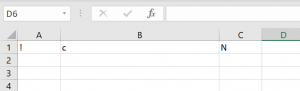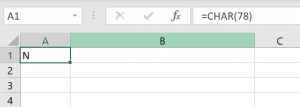### VBA Char / Chr Function

Every character in Excel has an assigned number code to itself. The Chr function in VBA returns a character for a given number (ASCII) code. In Excel, a similar function is the Char function. In this step-by-step tutorial, for all levels of Excel and VBA users, you will see how to use both functions.

## Using the Chr Function in VBA

As we already mentioned in the introduction, the Chr function takes a number (ASCII code) and returns a corresponding character. There are 255 ASCII codes, so you must take care to pass numbers 1-255 to the function. If you pass a number greater than 255, you will get an error in code execution.

Let’s look at the code for the Chr function:

In the example, we want to put characters for ASCII codes 33, 99 and 78 in cells A1, B1 and C1 respectively.Image 1. Using the Chr function in VBA

As you can see in Image 1, the character for ASCII code 33 is “!”, for 99 is “c” and for “78” is N.

## Using the Char Function in Excel

The Char function also returns a character for ASCII code, but this function does not exist in VBA.

You will see on similar example how to use the Char function in Excel to get a character for ASCII code 78, using this formula:Image 2. Using the Char function in Excel

In the cell A1, we want to get a character for ASCII code 78. As a result, the value of the cell A1 is “N”. The same result we got in the previous example, using the Chr function in VBA.

#### You may also like some of this related content...

VBA has many string functions that will allow you to manipulate and work with text
VBA Round The VBA Round Function rounds numbers to a specified number of digits. Syntax
While working in VBA, you often need to convert strings into lowercase, uppercase or proper
In this article, you will learn how to use the IsEmpty function in VBA to
The following UDF will return the formulae for a given cell reference: VBA Function to